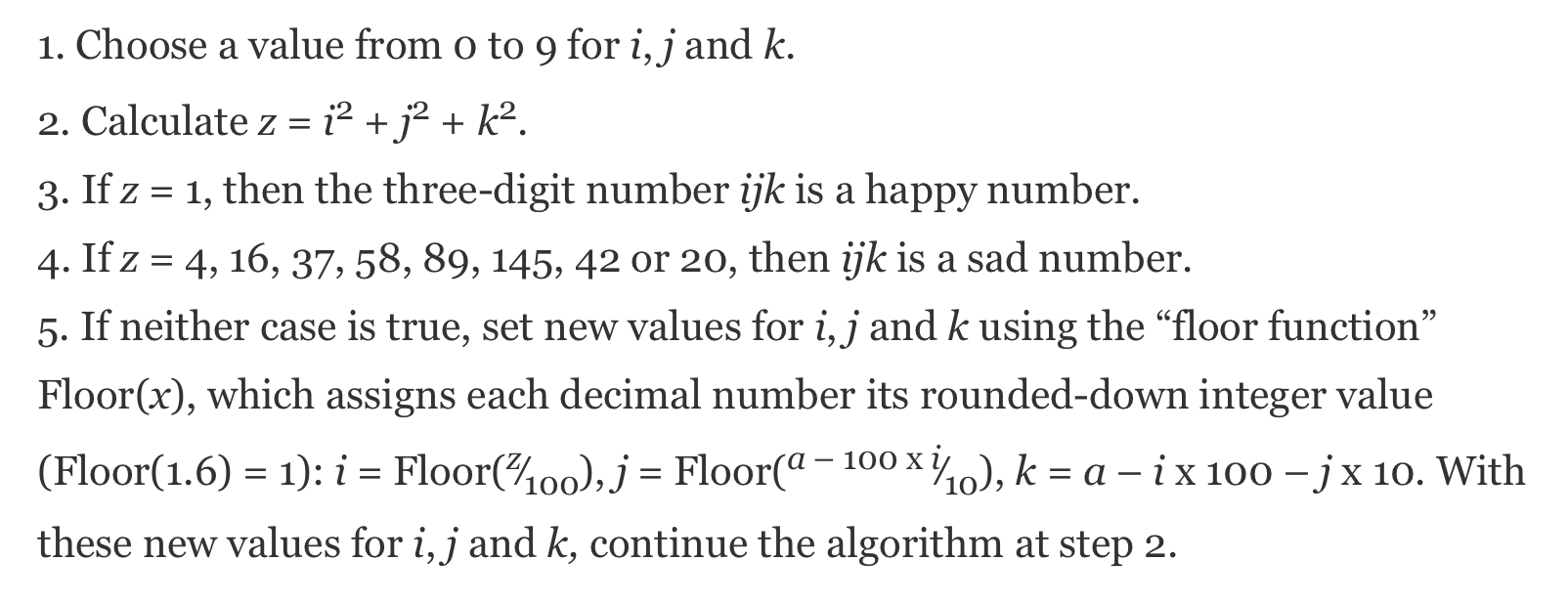Science writer Jennifer Ouillette linked to this Scientific American article on Mastodon this morning. It’s about happy and sad numbers, which I had never heard of before. The article is by Manon Bischof and was originally published in Spektrum der Wissenschaft, where she’s an editor.

Overall, the article made me happy, but one part of it made me sad. It’s this description of an algorithm you can use to categorize a three-digit number, where the hundreds, tens, and ones digits are i, j, and k, respectively:The problem is in Step 5 of the algorithm, where I’ve spread out the equations to make them easier to read:

5. If neither case is true, set new values for i, j and k using the “floor function” Floor(x), which assigns each decimal number its rounded-down integer value (Floor(1.6) = 1):

i = Floor(z100),

j = Floor(a – 100 x i10 ),

k = ai x 100 – j x 10.

With these new values for i, j and k, continue the algorithm at step 2.

What’s wrong with this? Let us count the ways:

1. The variable a in the equations for j and k should be z. There is no a.
2. The letter “x” should not be used to represent multiplication, especially when you’re using a serifed font, and especially especially when you’ve used it as a variable earlier in the sentence. There is a symbol for multiplication, ×, which has an easy-to-remember HTML entity, &times.
3. There’s no reason for a multiplication symbol in the first place. Surely Scientific American readers know that multiplication is implied when a number and a symbol are adjacent to one another: $100i=100×i$.
4. How about a little consistency in how you present multiplication? Yes, multiplication is commutative, but there’s no reason to write $100×i$ in one formula and $i×100$ in the next.
5. In fact, let’s go further. Always put the number first. No one typesets math with a variable before the number.

And although it’s not wrong, it might have helped readers to be more explicit in noting that these formulas are a mathematical way to extract the hundreds, tens, and ones digits from z (and any of these digits could be zero). In the examples earlier in the article, the digits were determined by inspection.

Because I’ve been thinking a lot about expressing formulas on the web lately, I decided to look at the HTML source for Step 5 in the SciAm article. Here it is:

xml:
5. If neither case is true, set new values for <em>i</em>,
<em>j</em> and <em>k</em> using the &ldquo;floor function&rdquo;
Floor(<em>x</em>), which assigns each decimal number its
rounded-down integer value (Floor(1.6) = 1):
<em>i</em> = Floor(<em><sup>z</sup></em>&frasl;<sub>100</sub>),
<em>j</em> = Floor(<em><sup>a</sup></em><sup> &ndash;
100 x <em>i</em></sup> &frasl; <sub>10</sub> ),
<em>k</em> = <em>a</em> &ndash; <em>i </em> x 100 &ndash;
<em>j</em> x 10.
With these new values for <em>i</em>, <em>j</em> and <em>k,</em>
continue the algorithm at step 2.


Using <sup> and <sub> is a reasonable approach for simple formulas like this, but why isn’t Scientific American using MathML, MathJax, or whatever other systems are out there for rendering mathematics on a web page? They’ve been printing mathematics in the hardcopy magazine for ages.

You may have noticed that I’ve switched over to MathML here.1 If you keep up with the blog via RSS, you’ve seen that posts with equations (like this one) have a disclaimer at the top, telling you that there are formulas in the post that your feed reader may not render correctly. If the formulas don’t render correctly, that’s probably because of the subscription service you’re using, not the reader. I intend to write a post explaining what I’ve learned about MathML, feed readers, and subscription services soon.

1. The MathJax logo in the sidebar and all the MathJax leftovers in the HTML source will be cleaned up as soon as I feel confident I’m done with all the changes.